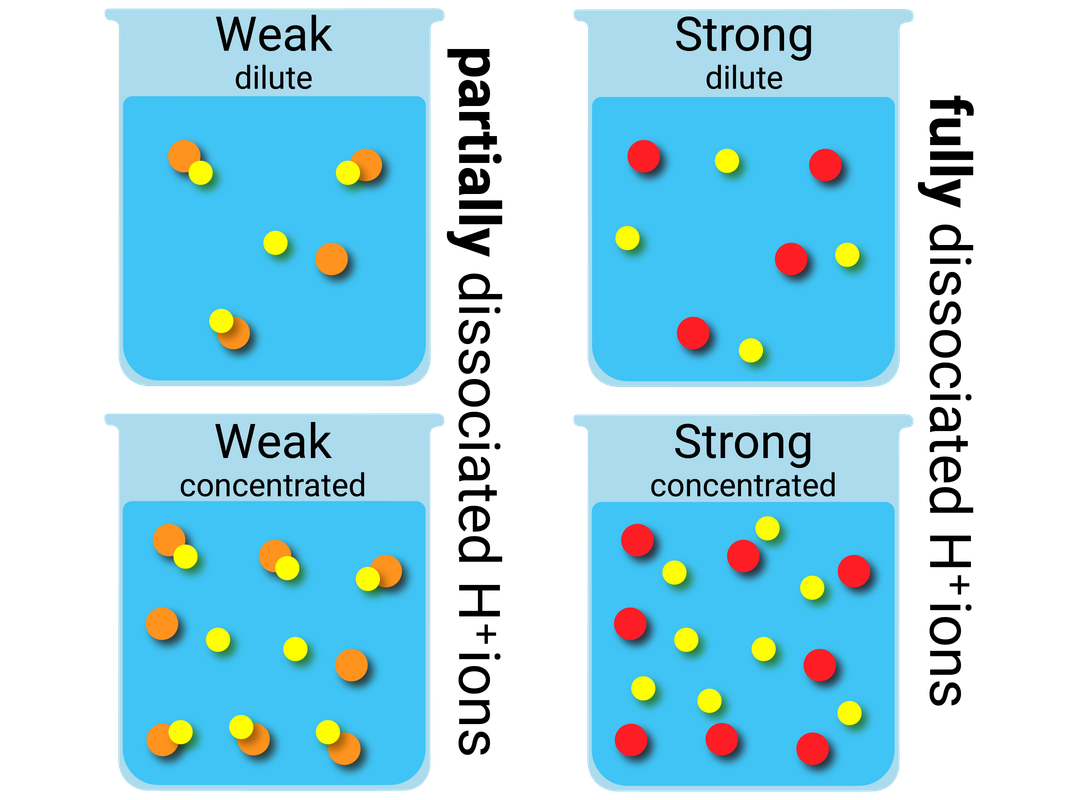# Types of Chemical Reaction

### REDOX (Reduction and Oxidation)

Some chemical reactions are classed as REDOX reactions. This is because a reduction, and oxidation reaction are happening at the same time.

When an element reacts with oxygen this is called oxidation.

When oxygen is removed from a compound, this is called reduction.

Higher Tier

When an ion loses electrons, this is called oxidation.

When an ion gains electrons,  this is called reduction.

### pH and Neutralisation

When universal indicator (UI) is added to solutions, it will change colour depending on what ions are present. UI can be used to estimate the pH of a substance using a colour chart, however pH probes give a much more accurate reading of pH.

The coloured pH scale below can only be used with indicators like UI, because these are the colours UI turns. Other indicators like methyl orange, and phenolphthalein, do not turn these colours - so this scale cannot be used.

 pH 1 pH 2 pH 3 pH 4 pH 5 pH 6 pH7 pH 8 pH 9 pH 10 pH 11 pH 12 pH 13 pH 14 strong acid acid weak acid neutral weak alkali alkali strong alkali

Acids are made up of hydrogen ions (H+), which are released when acids are dissolved in water. The more hydrogen ions there are in solution, the lower the pH of that solution.

A base is a substance that can neutralise an acid. The most common bases you will come across are metal oxides, metal hydroxides, and metal carbonates. Most bases are insoluble in water, and those that are soluble are called alkalis. Alkalis are made up of hydroxide ions (OH-).  When an acid and an alkali react, we can write an ionic equation to show how they make water:

H+(aq) + OH-(aq) → H2O(l)

When a base and an acid react, they produce a substance that is neutral. We call this a neutralisation reaction. A salt and water are always made during a neutralisation reaction.

acid + base → salt + water

### General Reactions

When acids react with different chemicals, they can make different products. Using different acids will create salts that have different names. In the table below you can see how each of these acids affects the name of the possible salt:

hydrochloric acid sulfuric acid nitric acid
salt name metal chloride metal sulfate metal nitrate

### Acids + Metals

A salt and hydrogen are produced when acids react with metals.

• acid + metal → salt + hydrogen
• hydrochloric acid + magnesium → magnesium chloride + hydrogen
• 2HCl(aq) + Mg(s) → MgCl2(aq) + H2(g)

### Acids + Metal Oxides

A salt and water are produced when acids react with metal oxides.

• acid + metal oxide → salt + water
• nitric acid + lithium oxide → lithium nitrate + water
• 2HNO3(aq) + Li2O(s) → 2LiNO3(aq) + H2O(l)

### Acids + Metal Hydroxides

A salt and water are produced when acids react with metal hydroxides.

• acid + metal hydroxide → salt + water
• sulfuric acid + sodium hydroxide → sodium sulfate + water
• H2SO4(aq) + 2NaOH(aq) → Na2SO4(aq) + 2H2O(l)

### Acids + Metal Carbonates

A salt, water, and carbon dioxide are produced when acids react with metal carbonates.

• acid + metal hydroxide → salt + water + carbon dioxide
• sulfuric acid + calcium carbonate → calcium sulfate + water + carbon dioxide
• H2SO4(aq) + CaCO3(s) → CaSO4(aq) + H2O(l) + CO2(g)

### Indicators

Indicators tells us what the pH is of a chemical by changing colour. Different indicators turn different colours based on what type of chemical is present.

We can also use technology, in the form of a pH meter to measure pH. These are more accurate and precise, when measuring pH, than indicators are. The pH meter may need calibrating first. This is done by adjusting the reading to match the pH of a buffer solution, which is a solution with a known pH.

### Titration Curves

When you carry out a simple acid-base titration, you use an indicator to tell you when you have the acid and alkali mixed in exactly the right proportions to "neutralise" each other. When the indicator changes colour, this is often described as the end point of the titration.

In an ideal world, the colour change would happen when you mix the two solutions together in exactly equation proportions. That particular mixture is known as the equivalence point.

We can run several experiments, with strong/weak acids vs alkalis, to show how easy it is to reach the equivalance point for each match.

Higher Tier

The higher the concentration of hydrogen ions in an acidic solution, the lower the pH.

The higher the concentration of hydroxide ions in an alkaline solution, the higher the pH.

As hydrogen ion concentration in a solution increases by a factor of 10, the pH of the solution will decrease by 1.

litmus paper methyl orange phenolphthalein
acid red red colourless
neutral purple orange colourless
alkali blue yellow pink/purple### Dilute/Concentrated and Weak/Strong

Higher Tier

solution forms when a solute dissolves in a solvent. The more concentrated the solution, the more particles it contains in a given volume.

When solutions are described as dilute or concentrated:

• a dilute solution contains a small amount of dissolved solute
• a concentrated solution contains a large amount of dissolved solute

Acids in solution are a source of hydrogen ions (H+). The hydrogen ions are produced when the acid dissociates (splits up) to form ions.

When acids are described as weak or strong:

• weak acids only partially dissociate into ions in solution, for example ethanoic acid, citric acid, and carbonic acid
• strong acids completely dissociate into ions in solution, for example hydrochloric acid, sulfuric acid, and nitric acid